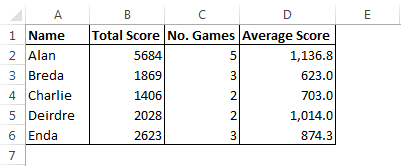# COUNTIF

The COUNTIF formula is another very useful formula in Excel for analysing data and getting more information from it.

The post on brackets and commas will show you how to break down longer formulas making them easier to read.  This post is also a continuation on earlier posts on IF statements and SUMIF formula.

## The story so far….

The SUMIF post left us with a file with 2 sheets, one sheet called “Scores” which lists scores achieved by players.The second sheet, called “Summary” gave us the total score achieved by each player.  I have added a 3rd column to this sheet, in this column we will show the number of games played by each player.## COUNTIF

Where the SUMIF formula add values in a column based on specific criteria, the COUNTIF formula counts how many times the specified criteria is met.

So far, our SUMIF tells us the total score achieved by each player, now we are going to work out how many times each person played the game.

The syntax of the COUNTIF formula is COUNTIF(RANGE, CRITERIA).

In Cell C2, our formula will be =COUNTIF(Scores!A:A,A2).  To breakdown this formula

• =COUNTIF( : This is a COUNTIF .formula and the parameters will be contained within the brackets.
• Scores!A:A : Our range is column A of the Scores sheet.  The range is where we are going to look for our criteria.
• A2 : Our criteria is the value in cell A2, that is, the players name.

In short, we want our formula to look for our name (criteria) in column A of the Scores sheet (range) and count how many times it appears.

Our Summary sheet now looks like this.Like the SUMIF formula, the COUNTIF formula can get very complicated looking, especially if the range is  in a different file that is closed.  Read over the SUMIF post for more on this, and remember as you read the formula to pay attention to the brackets and commas and use them to break down the formula, a short or long COUNTIF formula will the same syntax.

## Further Analysis

At this point we know the total score each person achieved and how many games they played.  By dividing the total score by the number of games we can get the average score from each player.

Simple formula like this can now open up a lot of options for further analysis of your data.